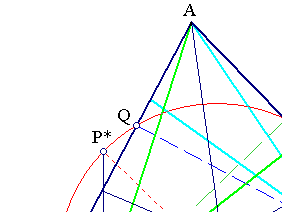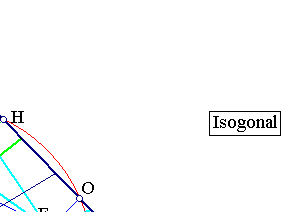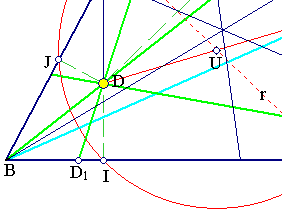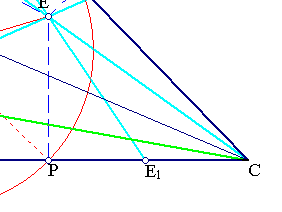##Isogonal Conjugation

Let t = (ABC) be a triangle. For each point D on its plane consider the symmetrics of DA, AB, AC with respect to the corresponding bisectors. The resulting lines EA, EB, EC concur at a point E, called the Isogonal-Conjugate of D.
The map f: D ---> E is the isogonal conjugation, defined by the triangle t.
f is involutive i.e. f2 = 1.The concurrence of lines at E can be proved easily using Ceva's theorem. For the trace D1 on BC it is
D1B/D1C=(DJ/sin(B))/(DH/sin(C)) = k1(sin(C)/sin(B)).
The corresponding trace E1 on BC has
E1B/E1C=(EQ/sin(B))/(EO/sin(C))=(1/k1)(sin(C)/sin(B)).
Later because of the symmetry on the bisector. By Ceva the product of the analogous ratios for all sides k1k2k3=1 implies that the corresponding ratios relative to E have also (1/k1)(1/k2)(1/k3)=1.
 The six projections of D and its isogonal E on the sides lie on a circle of radius r (the circumcircle of the triangle with vertices I, J, H, the projections of D on the sides of t). This is seen easily by considering the middle U of DE and identifying it with the center of the circle.
 Triangle t(D) = IJH is called the pedal triangle of point D with respect to triangle t. Its circumcircle c(U,r) is called the pedal circle of point D. Notice that triangles t(D), t(E) share the same pedal circle c(U,r).
 The symmetry of D, E on U implies that P*, diametral of P, has DP* parallel and equal to PE.
 This implies that |DI||EP| = r˛ - |DU|˛ = k (the power of D with respect to the circle), thus leading to the relation of the trilinear coordinates of E: (x*, y*, z*) = ( k/x, k/y, k/z ) to those of D.

The isogonal conjugation, given in trilinears by f : (x,y,z) -->(k/x, k/y, k/z), is not properly defined on the vertices of the triangle. Outside the sides (and their prolongations) f is one-to-one (invertible). f sends all the points of a side of the triangle to the opposite vertex.
The isogonal conjugation is a special case of the more general quadratic transforms related to families of conics (see IsogonalAsQuadratic.html ).
There are several applications of this transform. A non standard one can be found in the file: Isogonal_3TangentCircles.html .

Some remarkable triangle centers and their isogonal conjugates, {a,b,c} denoting the side-lengths of the triangle and {A,B,C} the angle-measures:

X1 : Incenter: (1,1,1) ----> Incenter, fixed point of isogonal conjugation.
X2 : Centroid: (1/a, 1/b, 1/c) ----> X6 : Symmedian point (a, b, c).
X3 : Circumcenter (cosA, cosB, cosC) ----> X4 : Orthocenter (1/cosA, 1/cosB, 1/cosC).
B1 : First Brocard point (c/b, a/c, b/a) ----> B2 : Second Brocard point (b/c, c/a, a/b).
X7 : Gergonne point (b*c/(b+c-a), ... ) ----> X55 : Internal center of similitude circum-/ in-circle (a*(b+c-a), ...).
X8 : Nagel point ((b+c-a)/a, ...) ----> X56 : External center of similitude circum-/ in-circle (a/(b+c-a),...).
The periods in the definitions of coordinates mean a cyclic permutation of {a,b,c}.